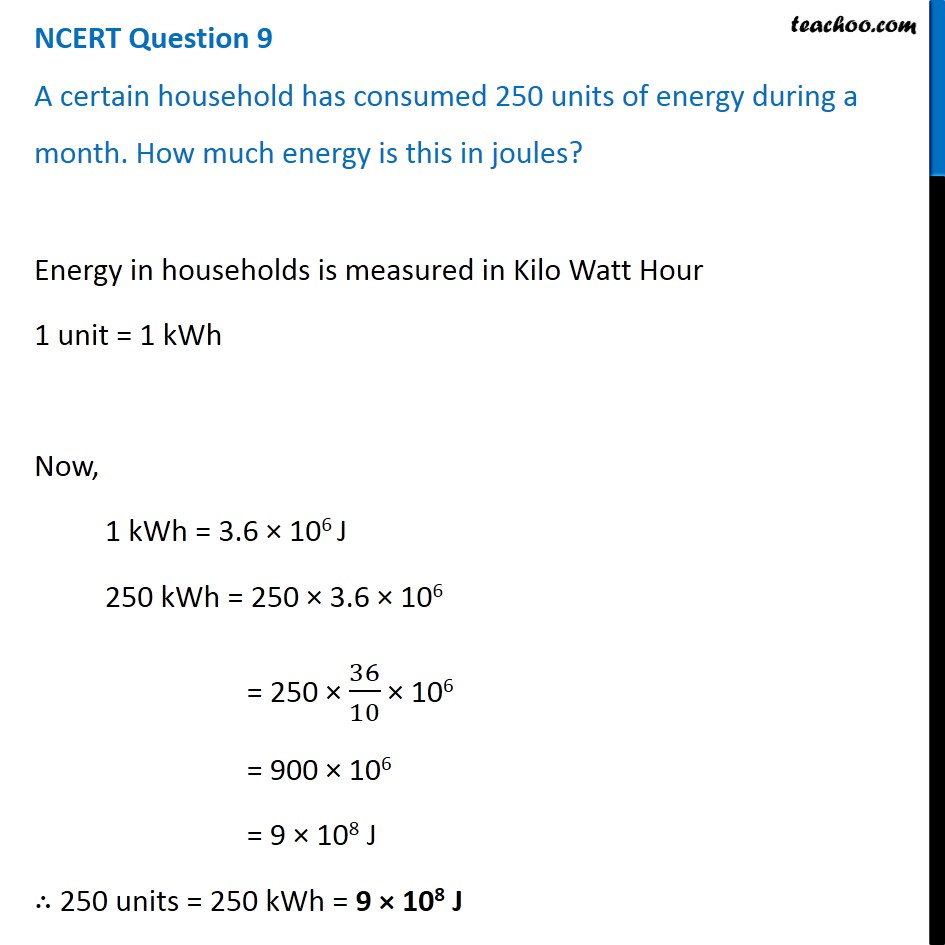1. Class 9
2. Chapter 11 Class 9 - Work and Energy (Term 2)
3. NCERT Questions

Transcript

NCERT Question 9 A certain household has consumed 250 units of energy during a month. How much energy is this in joules? Energy in households is measured in Kilo Watt Hour 1 unit = 1 kWh Now, 1 kWh = 3.6 × 106 J 250 kWh = 250 × 3.6 × 106 = 250 × 36/10 × 106 = 900 × 106 = 9 × 108 J ∴ 250 units = 250 kWh = 9 × 108 J

NCERT Questions

Class 9
Chapter 11 Class 9 - Work and Energy (Term 2)